# Master Quadratic Functions | Cambridge IGCSE Mathematics[Please watch the video attached at the end of this blog for a visual explanation on Master Quadratic Functions]

Quadratic functions are generally given in the format of ax2 + bx + c, where a, b and c would be different constants.

If the value of a is a positive value, then we would end up with a U-shaped curved graph or a parabola as shown below:We call these graphs minimum graphs because they have a minimum point as their vertex.

On the other hand if the value of a (coefficient of x2) is negative, then we would end up with an n-shaped graph, which is also known as a maximum curve.

It is known as a maximum curve because the vertex is a maximum value.

### How do we work out the equation of different quadratic functions when we are given different starting points?

If we have been given the vertex (either a maximum or minimum one) and one other point or one coordinate on our graph, how will we be able to find the equation of the quadratic function?

It is important to remember that when we have quadratic functions, we can write it using the standard format of y = ax2 + bx + c, and this is the standard form that we have, but we written in the form of y = a (x-h)2  k.  This format is also known as the completed square format, or the vertex form.

In vertex form, h gives us the x coordinate of our vertex (vx) and k gives us the y coordinate of our vertex (vy).

Example: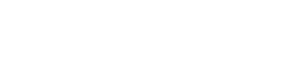1. We will first use the vertex form of the equation, and instead of h, we will substitute the value of x, which is 2 here, and instead of k, we will substitute the y value of our vertex which is 3.2. We then substitute the coordinate that we have been given.
The coordinate that we have been given is (3,8). Hence 3 would be substituted for x and 8 would be substituted for y. This would build up the equation as below:3. Once we have the value of a, we substitute the value of a and all the other values that we have already found out. We know h is 2, k is 3 and a is 5, therefore once we substitute these values into the equation, we will end up with: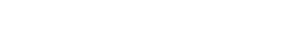4. We then expand the equation. We multiply 5 by (x – 2)2 and then add 3, resulting in the below value as the final answer: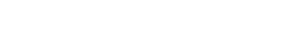As seen above, this equation is in the form of y = ax2 + bx + c, which is a standard form in which quadratic functions are given.

### How do we build up the equation of the quadratic function where we have been given the x-intercept and a point?

We know that the equation of a quadratic function would always be in the format of y = ax2 + bx + c, and we can factorise this to end up with the equation y = n(x + k) (x + h).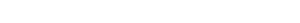If we look at the example they have given us, they have let us know that the x-intercepts are (-1, 0) and (3,0), and the coordinate of the other point is (2, -6).

Remember that we can also call our x-intercepts as the roots of our functions.

Therefore what this tells us is that if we look at x + k and x + h, both x + k and x + h would give us the value of zero at the first root and second root respectively.

If we look at the root that we have been given, the first root that we have been given is -1, therefore we will replace x with -1, and this means the value of k should be +1.

x + k = 0
-1 + k = 0
k = +1

Similarly, we can also work out the value of h.

x + h = 0
3 + h = 0
h = -3

This will then give us the equation as below:We also need to work the value of n. In order to do this, we need to substitute the second coordinate that we have been given (2, -6). Therefore instead of x we will substitute 2 and instead of y we will substitute -6in the above equation.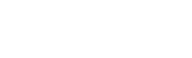Once we find out the value of n as being 2, we substitute all the values that we have found so far into the equation below:In order to present the equation in standard form (y = ax2 + bx + c), we can expand this equation.

Remember that when we expand two brackets, we have to multiply every term from the first bracket by every single term inside the second bracket.

This would result in the answer given below: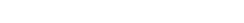We can then collect the like terms, forming our final equation: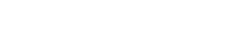### How do we build up the equation of a quadratic function if we have been given the vertex or the x-intercept?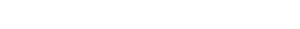Here we can see that they have not given this equation as y = ax2 + bx + c, they have just given y = x2 + bx + c, which indicates that the value of a = 1. They have also mentioned that the roots of this equation are -2,0 and 3,0.

Since we have been given the roots, we are going to look at the equation in the format of y = n (x + k) (x + h).

Because a = 1, we can say that n = 1, and we can therefore remove the n from the above equation. Therefore we have y = (x + k) (x + h). The value of k and h would just be the negative of the roots, therefore k = +2 and h = -3.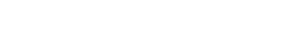Once we have this, we can expand the brackets and when we do that, it will give us the final answer once we collect the “like” terms together.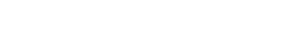Read through the questions and functions given carefully. Make sure that you recognise what is required of you and therefore you answer that specific question. Practise as many questions as you can on this topic. You can find some questions in this quiz to check where you stand!

If you are struggling with IGCSE revision or the Mathematics subject in particular, you can reach out to us at Tutopiya to join revision sessions or find yourself the right tutor for you.

Watch the video below for a visual explanation of the lesson on mastering quadratic functions.